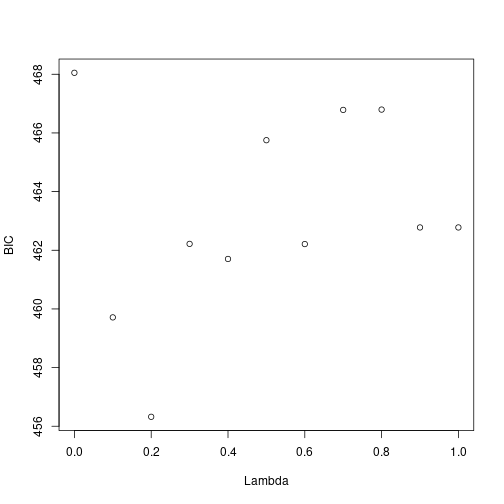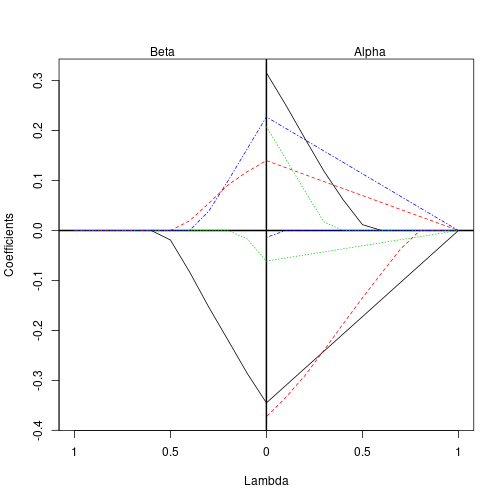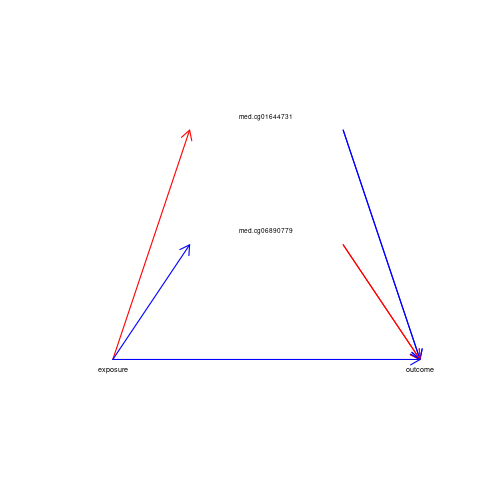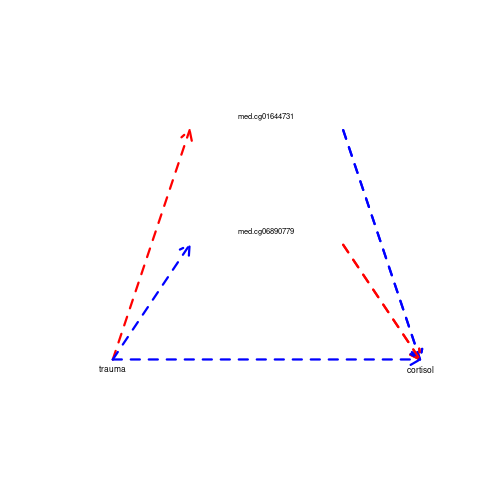# Overview

This is a demonstration of regularized mediation analysis (regmed) for mediation

The user user-level functions in the regmed package are:

• `regmed.grid()` penalized model for grid of lambdas
• `trim.best()` get best model, trim off mediators with no effect, refit model
• `regmed.fit()` fit penalized model with specified lambda
• `plot.regmed.grid()` plot results of regmed.grid
• `plot.regmed()` plot results of a single model fit, from either trim.best() or regmed.fit()
``````require("regmed")
``````

# Dataset

The data used in this example was created from selecting four methylyation loci as examples from the publicly available data E-GEOD-77445 - Genome-wide DNA methylation levels. The “exposure” variable is childhood trauma, the “outcome” variable is cortisol stress reactivity, and the four methylation loci are potential mediators to be evaluated.

This example data has only four potential mediators. In practice, the number of mediators can be much larger than the sample size. In this case, one can use sure independence screening (Fan, J., & Lv, J. , 2008, Sure independence screening for ultrahigh dimensional feature space. J. R. Statist. Soc.B, 70, 849-911). This approach is based on ranking marginal correlations and then selecting the highest ranked values such that the number of parameters is less than the sample size. Because mediation depends on the two correlations, r(x,m) and r(m,y), where m is a potential mediator, one can rank the absolute values of their products, |r(x,m) * r(m,y)|, and then select the highest ranked values to determine which potential mediators to include in the penalized mediation models, such that the number parameters in the model is less than the sample size.

``````data(regmed_example)

y <- regmed_example\$y
x <- regmed_example\$x
med <- regmed_example[, -c(1, 2)]
``````

# Fit Grid

Fit penalized models for a grid of lambda's

``````fit.grid <- regmed.grid(x, med, y, lambda.vec = c(seq(from = 1, to = 0, by = -0.1)),
frac.lasso = 0.8)
``````

## Plot regmed.grid object

``````plot.regmed.grid(fit.grid)
``````# Trim Best Model

Get best model, trim to remove mediators with near zero effect, and refit with specified lambda (default lambda = 0).

``````fit.trim <- trim.best(fit.grid)
summary.regmed(fit.trim)
``````
``````Call:
regmed.fit(x = x, mediator = med[, c("med.cg01644731", "med.cg06890779"),
drop = FALSE], y = y, lambda = 0, frac.lasso = 0.8)

Coefficients:
alpha       beta  alpha.beta
med.cg01644731  0.3186614 -0.3050835 -0.09721834
med.cg06890779 -0.3715008  0.2071779 -0.07696674

sum of alpha*beta =  -0.1741851
delta =  -0.1036191
sum of delta + alpha*beta =  -0.2778042
var(x) =  1
var(y) =  0.936029
``````

# Plot regmed object

``````plot(fit.trim, cex = 0.6)
``````# Fit regmed with different lambda penalties

This example subsets to the mediators in fit.trim, and uses regmed() on the selected subset of mediators.

``````## choose subset of mediators that are in fit.trim
which.med <- colnames(med) %in% dimnames(fit.trim\$alpha)[]
med.selected <- med[, which.med]
trauma = x
cortisol = y
fit.lam2 <- regmed.fit(trauma, med.selected, cortisol, lambda = 0.2, frac.lasso = 0.8)
summary(fit.lam2)
``````
``````Call:
regmed.fit(x = trauma, mediator = med.selected, y = cortisol,
lambda = 0.2, frac.lasso = 0.8)

Coefficients:
alpha       beta  alpha.beta
med.cg01644731  0.2029702 -0.1965572 -0.03989525
med.cg06890779 -0.2557726  0.1039875 -0.02659716

sum of alpha*beta =  -0.06649241
delta =  -0.1363947
sum of delta + alpha*beta =  -0.2028871
var(x) =  1
var(y) =  0.8880832
``````
``````fit.lam0 <- regmed.fit(trauma, med.selected, cortisol, lambda = 0, frac.lasso = 0.8)
summary(fit.lam0)
``````
``````Call:
regmed.fit(x = trauma, mediator = med.selected, y = cortisol,
lambda = 0, frac.lasso = 0.8)

Coefficients:
alpha       beta  alpha.beta
med.cg01644731  0.3186614 -0.3050835 -0.09721834
med.cg06890779 -0.3715008  0.2071779 -0.07696674

sum of alpha*beta =  -0.1741851
delta =  -0.1036191
sum of delta + alpha*beta =  -0.2778042
var(x) =  1
var(y) =  0.936029
``````

## Plot one of the fits from above

We show the plot method can display different sized text and line types, along with using the variable names from the call to regmed.fit().

``````plot(fit.lam2, lty = 2, lwd = 3, cex = 0.7)
``````# Use Lavaan to get SE of Parameters

When using trim.best() with default lambda = 0, the returned object is an unpenalized structural equation model fit. For this model, the lavaan R package can be used to estimate standard errors of the model parameters, although these are approximations that do not consider that the terms in the model were first chosen by the penalized model.

``````library(lavaan)
``````
``````This is lavaan 0.6-5
``````
``````lavaan is BETA software! Please report any bugs.
``````
``````## choose subset of mediators that are in fit.trim
which.med <- colnames(med) %in% dimnames(fit.trim\$alpha)[]
med.selected <- med[, which.med]
mediator.names <- dimnames(med.selected)[]

## setup lavaan model
med.model <- lavaan.model(y.name = "y", x.name = "x", med.name = mediator.names,
medcov = fit.trim\$MedCov)

## setup data for lavaan
dat <- data.frame(cbind(scale(x, center = TRUE, scale = TRUE), scale(y, center = TRUE,
scale = TRUE), scale(med.selected, center = TRUE, scale = TRUE)))
names(dat) <- c("x", "y", dimnames(med.selected)[])

## fit sem with lavaan

fit.lavaan <- sem(model = med.model, data = dat)
summary(fit.lavaan)
``````
``````lavaan 0.6-5 ended normally after 14 iterations

Estimator                                         ML
Optimization method                           NLMINB
Number of free parameters                          6

Number of observations                            85

Model Test User Model:

Test statistic                                 0.030
Degrees of freedom                                 3
P-value (Chi-square)                           0.999

Parameter Estimates:

Information                                 Expected
Information saturated (h1) model          Structured
Standard errors                             Standard

Regressions:
Estimate  Std.Err  z-value  P(>|z|)
y ~
x                -0.098    0.109   -0.894    0.372
med.cg01644731   -0.309    0.102   -3.029    0.002
med.cg06890779    0.211    0.104    2.029    0.042
med.cg01644731 ~
x                 0.319    0.103    3.083    0.002
med.cg06890779 ~
x                -0.371    0.101   -3.666    0.000

Covariances:
Estimate  Std.Err  z-value  P(>|z|)
.med.cg01644731 ~~
.med.cg06890779     0.000

Variances:
Estimate  Std.Err  z-value  P(>|z|)
.med.cg01644731    0.898
.med.cg06890779    0.862
.y                 0.793    0.122    6.519    0.000
``````

## Description of Lavaan Output

The output from lavaan sem model shows the regressions for y regressed on x and the mediators. The estimates and std.err for these coefficents are the direct effect of x on y (delta), and the the beta coeffients for the mediators. The regression of each mediator on x gives the estimate of alpha and its std.err. The covariances of the mediators are fixed (so no std.err), see reference by Schaid and Sinnwell (2020). The estimate of the residual variance of y is shown in the section of “Variances”, along with its std.err.

# References

• Schaid DJ, Sinnwell JP. (2020) Penalized Models for Analysis of Multiple Mediators. Genet Epidemiol, to appear.

• Rosseel Y. (2012) lavaan: An R Package for Structural Equation Modeling. Journal of Statistical Software, 48(2), 1–36. http://www.jstatsoft.org/v48/i02/.